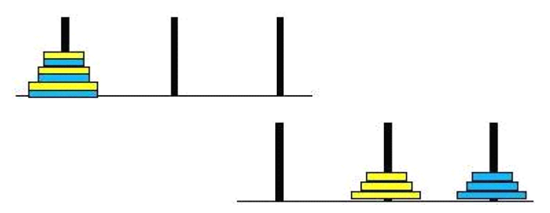# 借汉诺塔理解栈与递归

def hanoi(n, a, b, c):
if n == 1:
print(a, '-->', c)
else:
hanoi(n - 1, a, c, b)
print(a, '-->', c)
hanoi(n - 1, b, a, c)
# 调用
hanoi(3, 'A', 'B', 'C')

A --> C
A --> B
C --> B
A --> C
B --> A
B --> C
A --> C2, 'A', 'C', 'B'

3, 'A', 'B', 'C'

2, 'A', 'C', 'B'
3, 'A', 'B', 'C'

3, 'A', 'B', 'C'

def hanoi(n, a, b, c):
if n == 1:
print(a, '-->', c)
else:
hanoi(n - 1, a, c, b)//执行到了这里
print(a, '-->', c)
hanoi(n - 1, b, a, c)10-28
11-07226703-074万+
11-132760
04-294万+
07-214215
08-031万+
03-306682
05-011万+
03-132万+